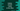# How to convert a python string to the hexadecimal value## How to convert a python string to hex value:

In this post, I will show you how to convert a python string to a hexadecimal value. The hexadecimal value starts with 0x. Python provides a method called hex to convert an integer to hexadecimal value.

For converting a string to hex value, we need to convert that string to an integer. Well, we have another method to convert a string to an integer in python as well. We can combine both of these methods to convert a string to hex.

### Convert string to integer:

We need to use int(str, 16) to convert a hexadecimal string to an integer. For example:

``````given_str = "0xAA"

print(int(given_str, 16))``````

If you run this program, it will print:

``170``

170 is the decimal value of 0xAA.

### Convert integer to hex value:

To convert an integer to hex, we can use hex(). For example,

``print(hex(170))``

It will print:

``oxaa``

That’s it. We can combine both of these approaches to convert a string to hex in python.

### Python program to convert a string to hex:

Let’s combine both of these methods and write the final python program to convert a string to hex :

``````given_string = '0xAA'

int_value = int(given_string, 16)

hex_value = hex(int_value)

print("Hex of {} is {}".format(given_string, hex_value))``````

It will print:

``Hex of 0xAA is 0xaa``

### Errors and exceptions:

The hex function throws a TypeError if the parameter is not an integer. For example:

``````given_value = '0xaa'

print(hex(given_value))``````

It will throw one TypeError:

``TypeError: 'str' object cannot be interpreted as an integer``